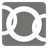Count Chains
Simple
English

In this mission you must count chains.

You are given a list of details of the circle (tuple of X-coordinate, Y-coordinate, radius).
You have to return the number of groups of circles where their circumferences intersect.

NOTE:

Only one circle counts as one group.

Example:

```count_chains([(1, 1, 1), (4, 2, 1), (4, 3, 1)]) == 2
count_chains([(1, 1, 1), (2, 2, 1), (3, 3, 1)]) == 1
count_chains([(1, 1, 1), (1, 3, 1), (3, 1, 1), (3, 3, 1)]) == 4
```Input:

• A list of details of the circle.
• Details of the circle is a tuple of three integers(X-coordinate, Y-coordinate, radius).

Output: An integer.

Precondition:

• -10 ≤ x(, y) coordinate ≤ 10
• 1 ≤ radius ≤ 10